Documentation

### This is machine translation

Mouseover text to see original. Click the button below to return to the English version of the page.

## Explicit MPC Control of a Single-Input-Single-Output Plant

This example shows how to control a double integrator plant under input saturation in Simulink® using explicit MPC.

### Define Plant Model

The linear open-loop dynamic model is a double integrator:

```plant = tf(1,[1 0 0]); ```

### Design MPC Controller

Create the controller object with sampling period, prediction and control horizons:

```Ts = 0.1; p = 10; m = 3; mpcobj = mpc(plant, Ts, p, m); ```
```-->The "Weights.ManipulatedVariables" property of "mpc" object is empty. Assuming default 0.00000. -->The "Weights.ManipulatedVariablesRate" property of "mpc" object is empty. Assuming default 0.10000. -->The "Weights.OutputVariables" property of "mpc" object is empty. Assuming default 1.00000. ```

Specify actuator saturation limits as MV constraints.

```mpcobj.MV = struct('Min',-1,'Max',1); ```

### Generate Explicit MPC Controller

Explicit MPC executes the equivalent explicit piecewise affine version of the MPC control law defined by the traditional MPC. To generate an Explicit MPC from a traditional MPC, you must specify range for each controller state, reference signal, manipulated variable and measured disturbance so that the multi-parametric quadratic programming problem is solved in the parameter space defined by these ranges.

Obtain a range structure for initialization

Use `generateExplicitRange` command to obtain a range structure where you can specify range for each parameter afterwards.

```range = generateExplicitRange(mpcobj); ```
```-->Converting the "Model.Plant" property of "mpc" object to state-space. -->Converting model to discrete time. Assuming no disturbance added to measured output channel #1. -->The "Model.Noise" property of the "mpc" object is empty. Assuming white noise on each measured output channel. ```

Specify ranges for controller states

MPC controller states include states from plant model, disturbance model and noise model in that order. Setting the range of a state variable is sometimes difficult when the state does not correspond to a physical parameter. In that case, multiple runs of open-loop plant simulation with typical reference and disturbance signals are recommended in order to collect data that reflect the ranges of states.

```range.State.Min(:) = [-10;-10]; range.State.Max(:) = [10;10]; ```

Specify ranges for reference signals

Usually you know the practical range of the reference signals being used at the nominal operating point in the plant. The ranges used to generate Explicit MPC must be at least as large as the practical range.

```range.Reference.Min = -2; range.Reference.Max = 2; ```

Specify ranges for manipulated variables

If manipulated variables are constrained, the ranges used to generate Explicit MPC must be at least as large as these limits.

```range.ManipulatedVariable.Min = -1.1; range.ManipulatedVariable.Max = 1.1; ```

Construct the Explicit MPC controller

Use `generateExplicitMPC` command to obtain the Explicit MPC controller with the parameter ranges previously specified.

```mpcobjExplicit = generateExplicitMPC(mpcobj, range); display(mpcobjExplicit); ```
``` Regions found / unexplored: 19/ 0 Explicit MPC Controller --------------------------------------------- Controller sample time: 0.1 (seconds) Polyhedral regions: 19 Number of parameters: 4 Is solution simplified: No State Estimation: Default Kalman gain --------------------------------------------- Type 'mpcobjExplicit.MPC' for the original implicit MPC design. Type 'mpcobjExplicit.Range' for the valid range of parameters. Type 'mpcobjExplicit.OptimizationOptions' for the options used in multi-parametric QP computation. Type 'mpcobjExplicit.PiecewiseAffineSolution' for regions and gain in each solution. ```

Use `simplify` command with the "exact" method to join pairs of regions whose corresponding gains are the same and whose union is a convex set. This practice can reduce memory footprint of the Explicit MPC controller without sacrifice any performance.

```mpcobjExplicitSimplified = simplify(mpcobjExplicit, 'exact'); display(mpcobjExplicitSimplified); ```
``` Regions to analyze: 15/ 15 Explicit MPC Controller --------------------------------------------- Controller sample time: 0.1 (seconds) Polyhedral regions: 15 Number of parameters: 4 Is solution simplified: Yes State Estimation: Default Kalman gain --------------------------------------------- Type 'mpcobjExplicitSimplified.MPC' for the original implicit MPC design. Type 'mpcobjExplicitSimplified.Range' for the valid range of parameters. Type 'mpcobjExplicitSimplified.OptimizationOptions' for the options used in multi-parametric QP computation. Type 'mpcobjExplicitSimplified.PiecewiseAffineSolution' for regions and gain in each solution. ```

The number of piecewise affine region has been reduced.

### Plot Piecewise Affine Partition

You can review any 2-D section of the piecewise affine partition defined by the Explicit MPC control law.

Obtain a plot parameter structure for initialization

Use `generatePlotParameters` command to obtain a parameter structure where you can specify which 2-D section to plot afterwards.

```params = generatePlotParameters(mpcobjExplicitSimplified); ```

Specify parameters for a 2-D plot

In this example, you plot the 1th state variable vs. the 2nd state variable. All the other parameters must be fixed at a value within its range.

```params.State.Index = []; params.State.Value = []; ```

Fix other reference signals

```params.Reference.Index = 1; params.Reference.Value = 0; ```

Fix manipulated variables

```params.ManipulatedVariable.Index = 1; params.ManipulatedVariable.Value = 0; ```

Plot the 2-D section

Use `plotSection` command to plot the 2-D section defined previously.

```plotSection(mpcobjExplicitSimplified, params); axis([-4 4 -4 4]); grid xlabel('State #1'); ylabel('State #2'); ```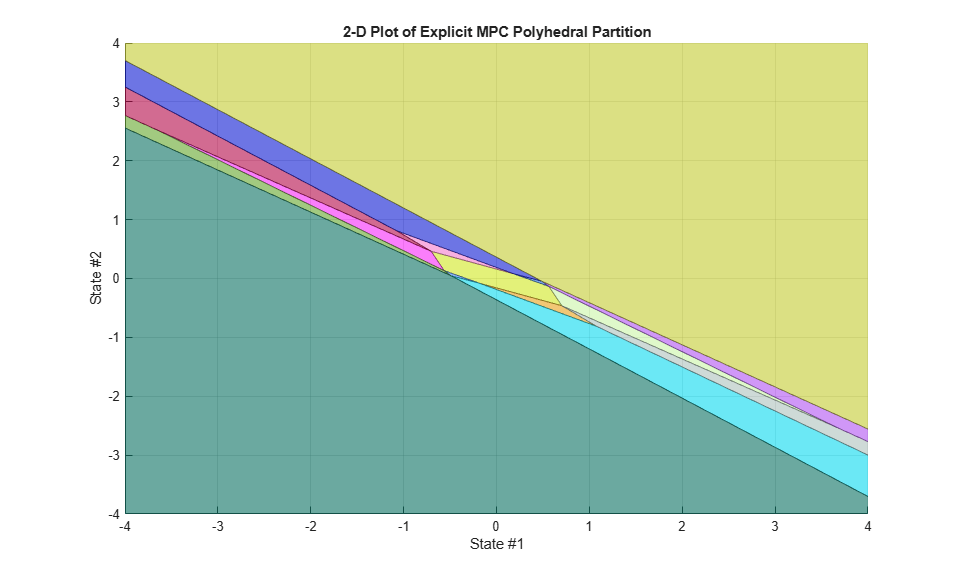### Simulate Using MPCMOVE Command

Compare closed-loop simulation between tradition MPC (as referred as Implicit MPC) and Explicit MPC using `mpcmove` and `mpcmoveExplicit` commands respectively.

Prepare to store the closed-loop MPC responses.

```Tf = round(5/Ts); YY = zeros(Tf,1); YYExplicit = zeros(Tf,1); UU = zeros(Tf,1); UUExplicit = zeros(Tf,1); ```

Prepare the real plant used in simulation

```sys = c2d(ss(plant),Ts); xsys = [0;0]; xsysExplicit = xsys; ```

Use MPCSTATE object to specify the initial states for both controllers

```xmpc = mpcstate(mpcobj); xmpcExplicit = mpcstate(mpcobjExplicitSimplified); ```

Simulate closed-loop response in each iteration.

```for t = 0:Tf % update plant measurement ysys = sys.C*xsys; ysysExplicit = sys.C*xsysExplicit; % compute traditional MPC action u = mpcmove(mpcobj,xmpc,ysys,1); % compute Explicit MPC action uExplicit = mpcmoveExplicit(mpcobjExplicit,xmpcExplicit,ysysExplicit,1); % store signals YY(t+1)=ysys; YYExplicit(t+1)=ysysExplicit; UU(t+1)=u; UUExplicit(t+1)=uExplicit; % update plant state xsys = sys.A*xsys + sys.B*u; xsysExplicit = sys.A*xsysExplicit + sys.B*uExplicit; end fprintf('\nDifference between traditional and Explicit MPC responses using MPCMOVE command is %g\n',norm(UU-UUExplicit)+norm(YY-YYExplicit)); ```
```Difference between traditional and Explicit MPC responses using MPCMOVE command is 1.69201e-13 ```

### Simulate Using SIM Command

Compare closed-loop simulation between tradition MPC and Explicit MPC using `sim` commands respectively.

```Tf = 5/Ts; % simulation iterations [y1,t1,u1] = sim(mpcobj,Tf,1); % simulation with tradition MPC [y2,t2,u2] = sim(mpcobjExplicitSimplified,Tf,1); % simulation with Explicit MPC ```
```-->Converting the "Model.Plant" property of "mpc" object to state-space. -->Converting model to discrete time. Assuming no disturbance added to measured output channel #1. -->The "Model.Noise" property of the "mpc" object is empty. Assuming white noise on each measured output channel. ```

The simulation results are identical.

```fprintf('\nDifference between traditional and Explicit MPC responses using SIM command is %g\n',norm(u2-u1)+norm(y2-y1)); ```
```Difference between traditional and Explicit MPC responses using SIM command is 1.69168e-13 ```

To run this example, Simulink® is required.

```if ~mpcchecktoolboxinstalled('simulink') disp('Simulink(R) is required to run this example.') return end ```

Simulate with traditional MPC controller in Simulink. Controller "mpcobj" is specified in the block dialog.

```mdl = 'mpc_doubleint'; open_system(mdl); sim(mdl); ```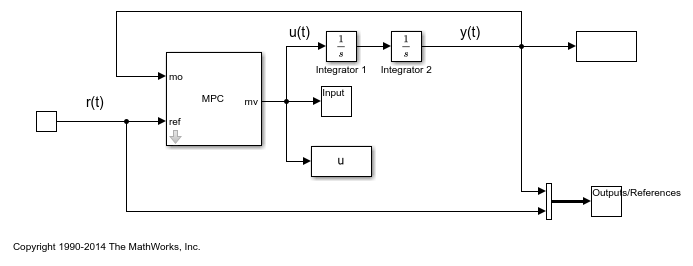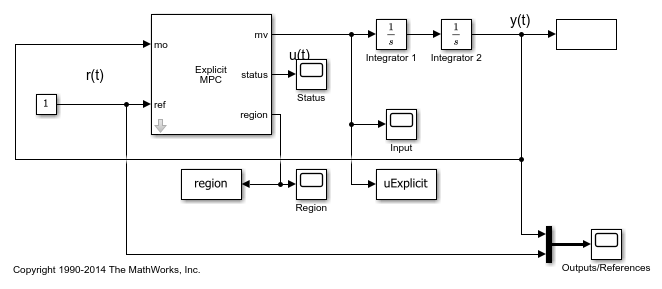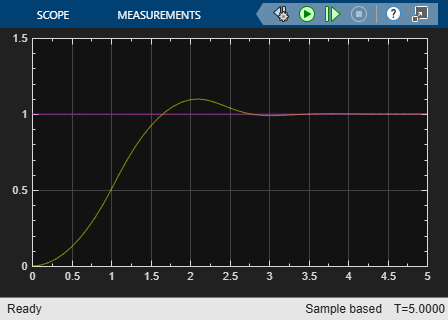Simulate with Explicit MPC controller in Simulink. Controller "mpcobjExplicitSimplified" is specified in the block dialog.

```mdlExplicit = 'empc_doubleint'; open_system(mdlExplicit); sim(mdlExplicit); ```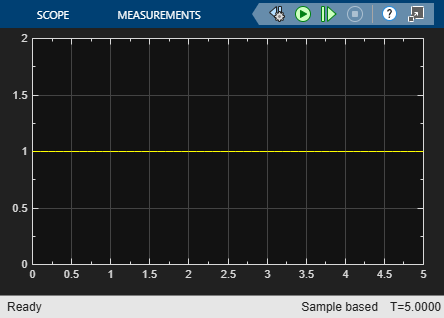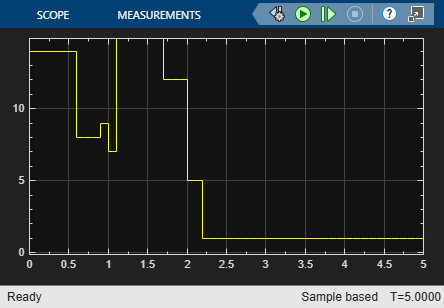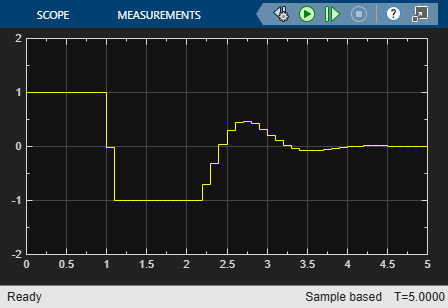The closed-loop responses are identical.

```fprintf('\nDifference between traditional and Explicit MPC responses in Simulink is %g\n',norm(uExplicit-u)+norm(yExplicit-y)); ```
```Difference between traditional and Explicit MPC responses in Simulink is 1.67292e-13 ```
```bdclose(mdl) bdclose(mdlExplicit) ```